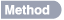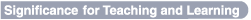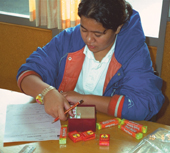DIVIDING UP THE PIZZA? A CONTEXT FOR ASSESSING FRACTIONSGlenda Anthony and Margaret Walshaw

 Manipulative and representational models of mathematical concepts create a reference framework through which abstract mathematical knowledge and procedures can be introduced, exemplified and understood in the classroom. Analysis of a NEMP task involving a contextually based ‘sharing’ problem that used circular representations of pizzas enabled an exploration of the influence of children’s informal knowledge on their developing understanding of fractions.The videotaped responses of 60 Year 4 and 60 Year 8 students to five questions were studied. Two model pizzas (one ham/one pepperoni) cut into sections of four and set on plates were provided.

The questions were:
1. How much of the pepperoni pizza is left?
2. How much of the ham and pineapple is left?
3. All together, how much pizza is left?
4. Let’s now think about two different ways of using up the pizza that’s left over.
If four children had a quarter piece of pizza each, how much would be left?
Prompt, if answer not given as fraction: What fraction or part is left?

Year 8 students were asked two extra questions:

1. Now imagine that the two of us are going to have an equal share of the pizza that is left.
What fraction or part of a whole pizza do we each get?
2. Can you explain to me how you worked that out?
Prompt: You can move the pieces of pizza around to help you work it out.• Fractional understanding builds incrementally, over considerable time, rather than being an all-or-nothing occurrence and is very much a function associated with educational experiences. • Context played a significant part for Year 4 students, with about 12% of the answers to Q1 and Q2 revolving around the orientation or specific toppings of the pizza. • About one quarter of Year 4 students responded with a natural number response to Q1 and Q2. • There was marked development between the age groups in understanding part/whole distributions (81% versus 35%). • Few Year 4 students exhibited knowledge of fraction addition of parts greater than one (Q3). Ten percent of Year 4 students adequately dealt with the whole pizza but could not name the remaining fraction. • Year 8 students solved the sharing questions by physically sharing the pieces, division by 2, halving, or estimation. Most students’ preferred approach involved referencing whole number partitioning strategies rather than more formal fraction operations.The findings emphasise the important role of students’ contextual and informal knowledge. For Year 4 students who have yet to receive extensive ‘formal’ instruction about fractions, informal knowledge dominated their solution strategies. Their solutions were more often dependent on the situation with its concrete and visual supports, rather than on symbolic manipulation. Year 8 students were more able to mathematise the problem situation, generally using mathematical language to provide an answer. However, some Year 8 students demonstrated a lack of ‘operation sense’ with regards to fractions. Their formal knowledge took precedence over their informal, and for those students who relied on partially constructed and remembered algorithms, mathematically non-sensible answers were proffered. Developing a productive mathematical disposition requires frequent opportunities for students to experience the rewards of sense-making in mathematics. Unless children are given sufficient opportunity to ‘make sense’ of realistic problem examples in appropriate contexts, they are unlikely to connect their informal knowledge of rational number concepts to their knowledge of formal symbols and procedures, or develop flexible understandings of fractions.